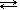# 3.5 The Common Ion Effect

$$\newcommand{\vecs}{\overset { \rightharpoonup} {\mathbf{#1}} }$$ $$\newcommand{\vecd}{\overset{-\!-\!\rightharpoonup}{\vphantom{a}\smash {#1}}}$$$$\newcommand{\id}{\mathrm{id}}$$ $$\newcommand{\Span}{\mathrm{span}}$$ $$\newcommand{\kernel}{\mathrm{null}\,}$$ $$\newcommand{\range}{\mathrm{range}\,}$$ $$\newcommand{\RealPart}{\mathrm{Re}}$$ $$\newcommand{\ImaginaryPart}{\mathrm{Im}}$$ $$\newcommand{\Argument}{\mathrm{Arg}}$$ $$\newcommand{\norm}{\| #1 \|}$$ $$\newcommand{\inner}{\langle #1, #2 \rangle}$$ $$\newcommand{\Span}{\mathrm{span}}$$ $$\newcommand{\id}{\mathrm{id}}$$ $$\newcommand{\Span}{\mathrm{span}}$$ $$\newcommand{\kernel}{\mathrm{null}\,}$$ $$\newcommand{\range}{\mathrm{range}\,}$$ $$\newcommand{\RealPart}{\mathrm{Re}}$$ $$\newcommand{\ImaginaryPart}{\mathrm{Im}}$$ $$\newcommand{\Argument}{\mathrm{Arg}}$$ $$\newcommand{\norm}{\| #1 \|}$$ $$\newcommand{\inner}{\langle #1, #2 \rangle}$$ $$\newcommand{\Span}{\mathrm{span}}$$

The common ion effect is another example of Le Châtelier's Principle (Unit 3 Equilibrium: Section 3.1) in action.

The common ion effect tells us that the solubility of an ionic compound is decreased by the addition to the solution of another ionic compound that contains one of the ions involved in the solution equilibrium.

Whew! That sounds a little confusing, but an example should help clarify what happens.

If we have a barium sulfate solution, the solid salt is in equilibrium with its ions:

 BaSO4 (s)Ba2+(aq) + SO42-(aq)

If we then add solid barium chloride to this solution, which dissolves to produce Ba2+ and Cl- ions, we are increasing the concentration of Ba2+ ions in our solution. (The new Cl- ions will remain in solution as spectator ions). Ba2+ is the ion common to both solutions.

Le Châtelier's Principle tells us that if the concentration of one of the reaction participants is increased, then equilibrium will shift to use up the additional substance.

So adding more Ba2+ will force the equilibrium to shift to the left (the reverse direction) in order to use up the added Ba2+ ions, producing more solid BaSO4. The concentration of SO42- will decrease, indicating that solubility has decreased.

3.5 The Common Ion Effect is shared under a not declared license and was authored, remixed, and/or curated by LibreTexts.